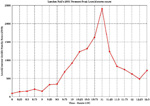## C Precedence Table

[chongo's home] [Astronomy] [Mathematics] [Prime Numbers] [Programming] [Technology] [contacting Landon]

The follow is the order of precedence, from highest to lowest, for the C programming language:

Operator Associativity
(expr)    [index]    ->    . Left ==> Right
!    ~    ++    --    (type)    sizeof
Unary operator:    +    -    *    &
Right <== Left
*    /    % Left ==> Right
+    - Left ==> Right
<<    >> Left ==> right
<    <=    >    >= Left ==> Right
==    != Left ==> Right
Binary operator:    & Left ==> Right
Binary operator:    ^ Left ==> Right
Binary operator:    | Left ==> Right
&& Left ==> Right
|| Left ==> Right
expr ? true_expr :  false_expr Right <== Left
+=    -=    *=    /=    <<=
&=   ^=    |=   %=   >>=    =
Right <== Left
, Left ==> Right
Unary
Operator
Example
+ +23209
- -value
* *pointer
& &variable
Binary
Operator
Example
& t = 0xCC; p = 0xAA;
(t & p) == 0x88;
^ r = 0xF0; w = 0xCC;
(r ^ w) == 0x3C;
| x = 0x99; y = 0x96;
(x | y) == 0x9F;

 © 1994-2013 Landon Curt Noll chongo (was here) /\oo/\ \$Revision: 7.2 \$ \$Date: 2012/05/14 08:52:26 \$